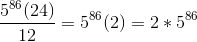## Example Questions

### Example Question #1 : How To Find Patterns In Exponents

For which of the following values of n will (3)n  represent a real number between 0 and 1?

1

2

0

2

1

2

Explanation:

In order to transform a negative integer to a positive one, it must be taken to an even power, and to make it a fraction between 0 and 1, it must be taken to a negative power. The only choice that fits both criteria is -2.

### Example Question #1 : How To Find Patterns In Exponents

A professional football player’s contract states that he will earn a salary of $1 million his first year. He would then have a 15% increase every year thereafter for the next 5 years. What would he make in his 6th and final season on the contract? Possible Answers: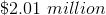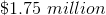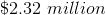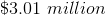Correct answer:Explanation: We can represent this as an exponential equation (just use million as a label and not a variable):$1m * (1.15)5 = \$2.01m

### Example Question #3 : How To Find Patterns In Exponents

What is the value of the expression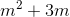, given that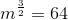?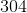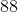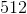Explanation:

First, calculate the value of m by taking the two-thirds root of both m3/2 and 64, leaving m=16. Then solve the expression by plugging in 16 for m. This gives 162 + 3(16) = 256 + 48 = 304.

### Example Question #4 : How To Find Patterns In Exponents

Evaluate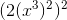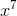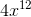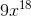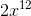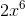Explanation: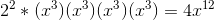### Example Question #261 : Exponents

If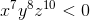, then which of the following must also be true?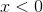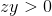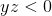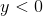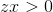Explanation:

We know that the expression must be negative. Therefore one or all of the terms x7, y8 and z10 must be negative; however, even powers always produce positive numbers, so y8 and z10 will both be positive. Odd powers can produce both negative and positive numbers, depending on whether the base term is negative or positive. In this case, x7 must be negative, so x must be negative. Thus, the answer is x < 0.

### Example Question #4 : How To Find Patterns In Exponents

Simplify the following: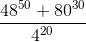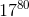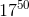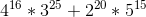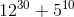Explanation:

With problems like this, it is always best to break apart your values into their prime factors. Let's look at the numerator and the denominator separately:

Numerator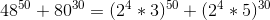Continuing the simplification: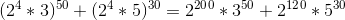Now, these factors have in common a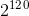. Factor this out: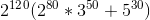Denominator

This is much simpler: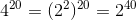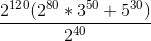Cancel out the common factors of: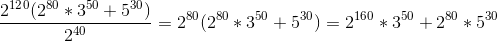### Example Question #1 : How To Find Patterns In Exponents

What digit appears in the units place when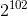is multiplied out?Explanation:

This problem is quite simple if you recall that the units place of powers of 2 follows a simple 4-step sequence.

Observe the first few powers of 2:

2= 2, 2= 4, 2= 8, 2= 16, 2= 32, 2= 64, 2= 128, 2= 256 . . .

The units place follows a sequence of 2, 4, 8, 6, 2, 4, 8, 6, etc. Thus, divide 102 by 4. This gives a remainder of 2.

The second number in the sequence is 4, so the answer is 4.

### Example Question #21 : Algebra

Which of the following is a multiple of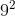?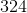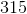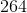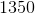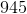Explanation:

For exponent problems like this, the easiest thing to do is to break down all the numbers that you have into their prime factors. Begin with the number given to you: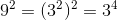Now, in order for you to have a number that is a multiple of this, you will need to have at least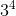in the prime factorization of the given number.  For each of the answer choices, you have: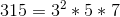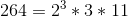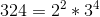; This is the answer.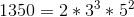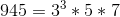### Example Question #1 : How To Find Patterns In Exponents

Simplify the following: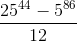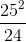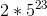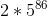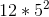Explanation:

Because the numbers involved in your fraction are so large, you are going to need to do some careful manipulating to get your answer. (A basic calculator will not work for something like this.) These sorts of questions almost always work well when you isolate the large factors and notice patterns involved. Let's first focus on the numerator. Go ahead and break apart theinto its prime factors: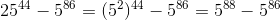Note that these have a common factor of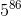. Therefore, you can rewrite the numerator as: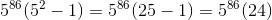Now, put this back into your fraction: